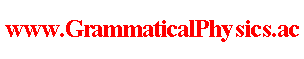since 2006 Help　Sitemap < Forum > < Products > < Quantum History Theory > < Physical Meaning of Uda Constant > I name α Uda constant. From Stacked Daruma Game Formula, I learned that 1/α seems to be a time length by which a quantum state is defined. Daruma formula suggests that there is no quantum state at an infinitely short moment. ν(t) is a quantum variable and has no counterpart in classical mechanics. This may mean that Energy Representation of Uda Equation (1) is not valid. However, Daruma formula perhaps teaches us some physics because it does not use time shorter than 1/α. It may be that the old quantum mechanics is a phenomenology of my quantum history theory at rough time scale. Here I write another aspect. Let us consider about Φ[ν] = exp{α∫-∞∞dt [-iωtν(t) + aν(t) + ibν(t)]}. I assume that | Φ[ν] |2 represent something proportional to something like probability. | Φ[ν] |2 = exp{α∫-∞∞dt [2aν(t)]}. [⊿/⊿ν(t)] | Φ[ν] |2 = 2α[aν(t)+1 - aν(t)] | Φ[ν] |2 = α ln[ |λν(t)+1|2 / |λν(t)|2 ] | Φ[ν] |2. [ | Φ[ν] |2 + ε[⊿/⊿ν(t)] | Φ[ν] |2 ] / | Φ[ν] |2 = 1 + εα ln[ |λν(t)+1|2 / |λν(t)|2 ]. ε must be greater than 1/α and must be very small. This is how much probability increases when we add +1 to the value of ν from t to t + ε. When Φ[χ] = exp[α∫-∞∞dt φ(χ(t), t)], is the wave function in the old grammar ψ(x, t) = exp[φ(x, t)]? If ∀t; ∀x; a ＜ t ＜ a + 1/α ⇒ (∂/∂t)φ(x, t) = 0, ∀t; ∀x; a ＜ t ＜ a + 1/α ⇒ ψ(x, t) = exp[φ(x, t)]. If ∃t; ∃x; a ＜ t ＜ a + 1/α and (∂/∂t)φ(x, t) ≠ 0, not ∀t; ∀x; a ＜ t ＜ a + 1/α ⇒ ψ(x, t) = exp[φ(x, t)]. I came to assume so by seeing Exact Solution of Uda Equation (6). --- The content of this page was developed in Number Representation of Uda Equation @ Quantum History Theory @ Problems. Author Yuichi Uda, Write start at 2019/11/10/17:36JST, Last edit at 2019/12/07/16:48JST